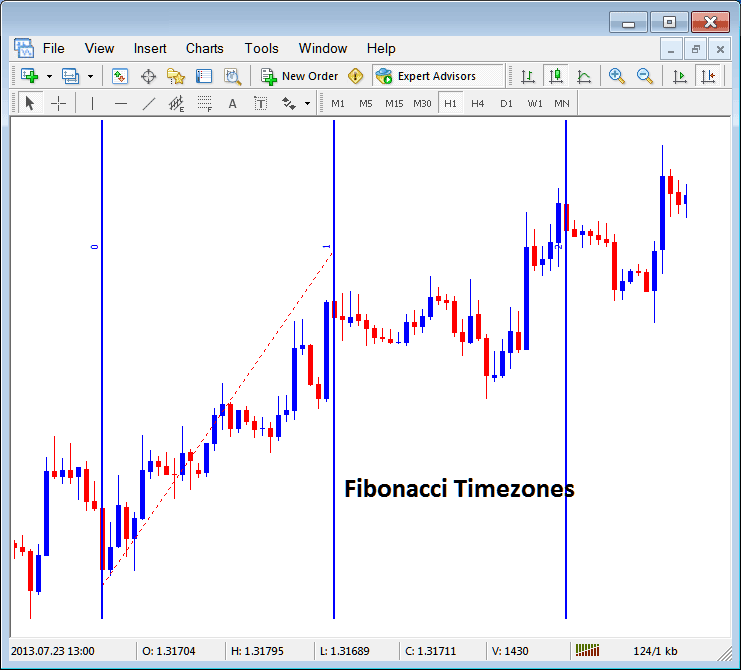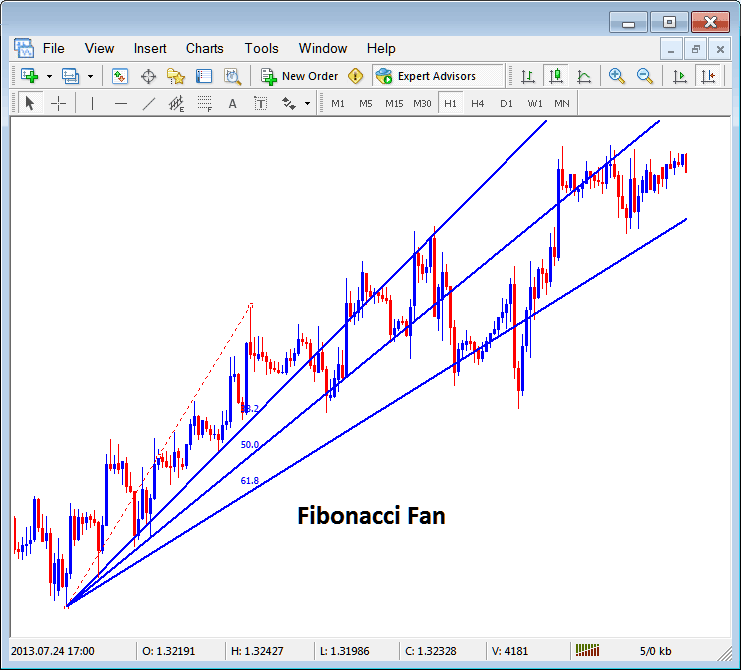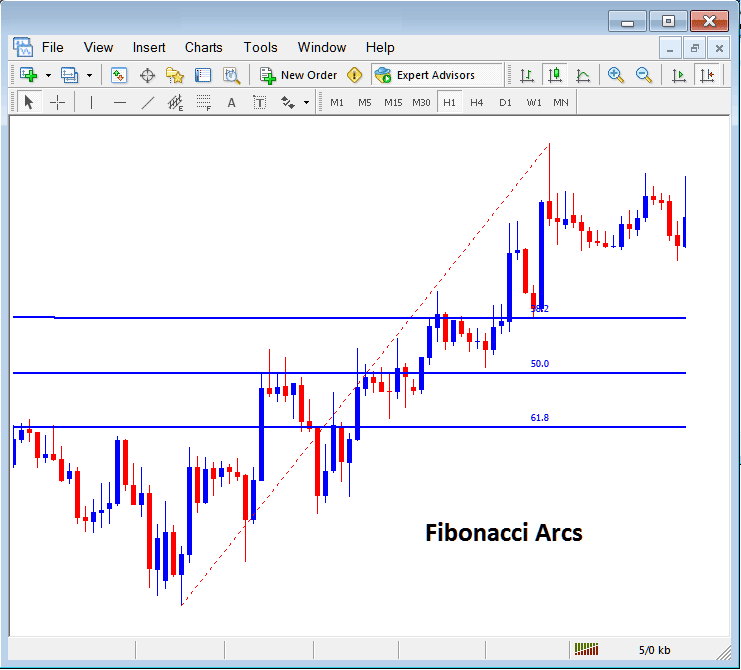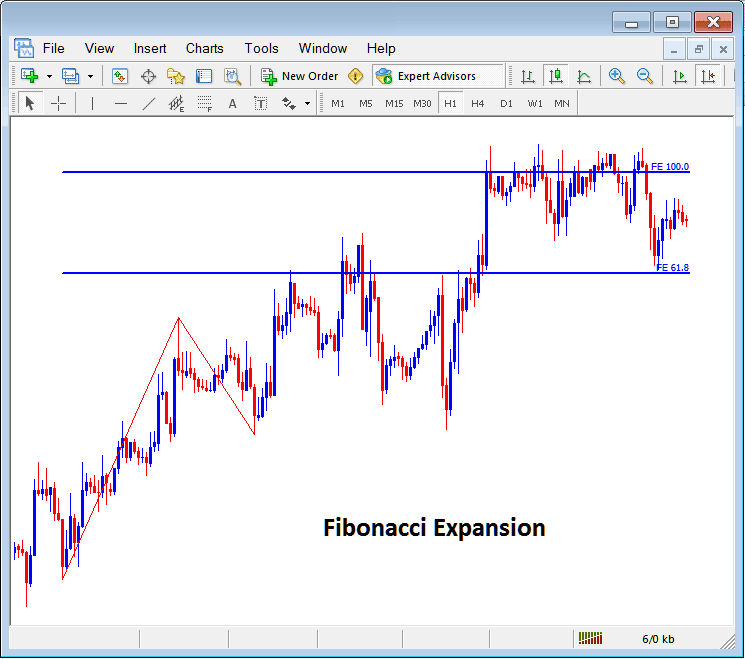# Placing Fibonacci Lines On MetaTrader 4 Forex Platform

The fifth option on the MetaTrader 4 Forex platform insert menu is Fibonacci Lines. The Fibonacci Lines Button has the following sub menu buttons shown below:## Fibonacci Lines

1. Fibonacci Retracement
2. Fibonacci  Time Zones
3. Fibonacci  Fan
4. Fibonacci  Arcs
5. Fibonacci  Expansion

## Fibonacci Retracement

The Fibonacci retracement can be placed on the a Forex chart from the Fibonacci retracement button. Fibonacci retracement is used to estimate where a price retracement is likely to reach. The Fibonacci retracement levels are drawn as shown below. To learn how to draw and use Fibonacci Retracement Levels read the tutorial: How to draw Fibonacci Retracement Levels:Fibonacci Retracement Levels Drawn on a Forex Chart on The MetaTrader 4 Forex Trading Platform

## Fibonacci Time Zones

The Fibonacci time zones are Fibonacci lines drawn at different Fibonacci timelines, these Fibonacci time zones are calculated using the Fibonacci points 1, 2, 3, 5, 8, 13, 21, 34. The Fibonacci levels are 1+2=3, 2+3=5, 3+5=8, first number add to the next, the next added to the second and so on.

The Fibonacci time zones are shown below, at these time lines price changes are likely to occur based on technical analysis of Fibonacci time zones:Fibonacci Time Zones on MT4 Platform

## Fibonacci Fan

The Fibonacci fan is drawn between two chart points and then three additional lines below the trend are drawn as the fans, these are 38.2%, 50.0% and 61.8% Fibonacci retracement levels. The price is likely to bounce and continue in the original direction after price retraces to any of these fan lines.

The Fibonacci Fan Lines are drawn as shown below:## Fibonacci Arcs

Fibonacci arcs are drawn using two chart points, the arcs are then drawn as shown below and these arcs represent 38.2%, 50.0% and 61.8% Fibonacci retracement levels.Fibonacci Arcs Representing Fibonacci Retracement Zones on a Forex Chart

## Fibonacci Expansion

The Fibonacci expansion is used to determine the most probable level at which traders should set their take profit levels based on Fibonacci expansion level 61.8% and 100.0% expansion levels. The Fibonacci expansion levels are also referred to as Fibonacci Extension Levels or Fibonacci Projection Levels by some traders and these Fibonacci Extension levels are drawn as shown below. Tutorial: How to Draw Fibonacci Expansion Levels:How to draw Fibonacci Lines and other Fibonacci studies like Fibonacci Expansion and Retracement on MetaTrader 4 FX Platform

Limited Time 100% Account Opening Bonus - Open Account

Top 100 Forex Broker Rankings